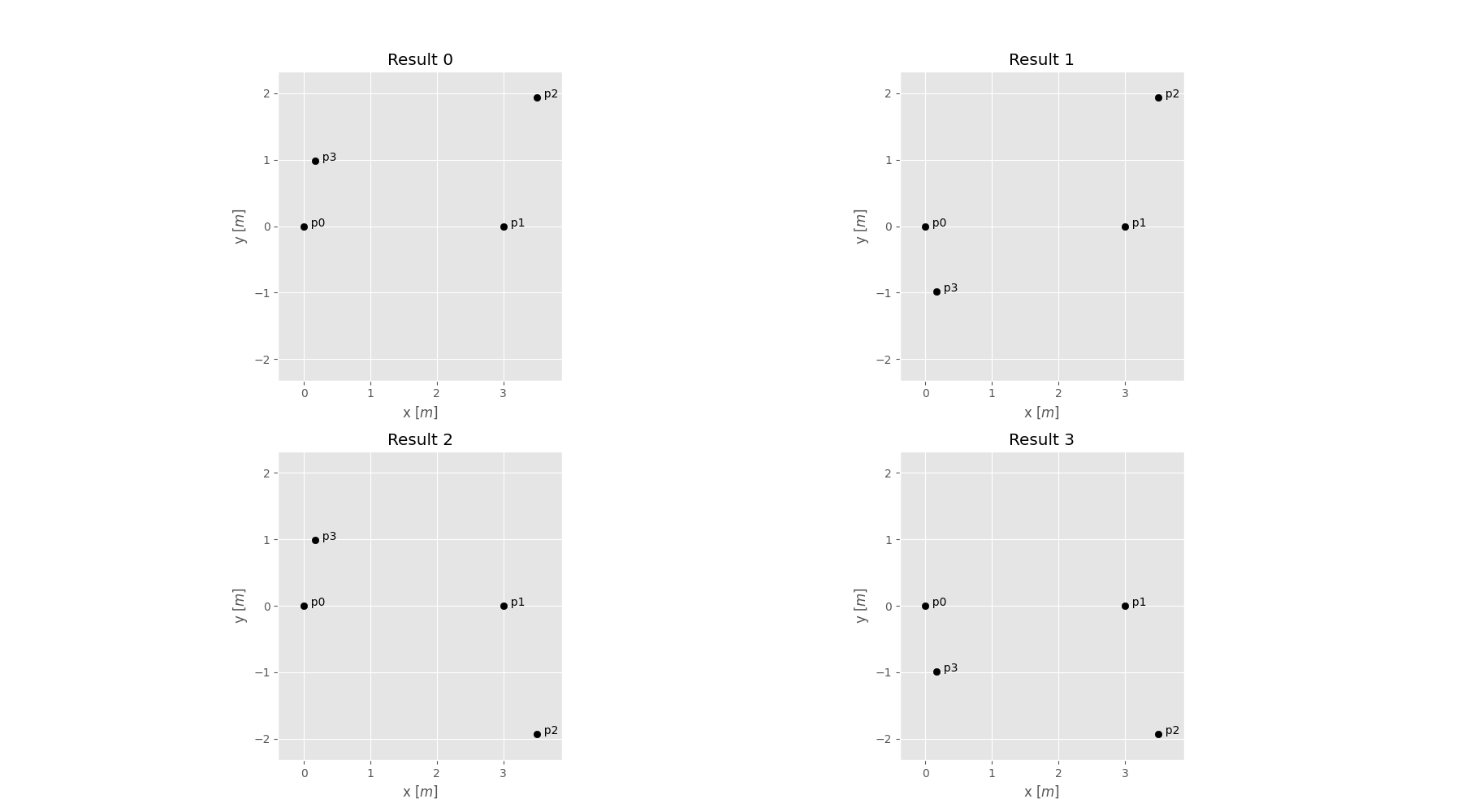# Point triangulation¶

Often, we only know the distances between wells within a well base field campaign. To retrieve their spatial positions, we provide a routine, that triangulates their positions from a given distance matrix.

If the solution is not unique, all possible constellations will be returned.

```import numpy as np
from welltestpy.tools import triangulate, sym, plot_well_pos

dist_mat = np.zeros((4, 4), dtype=float)
dist_mat[0, 1] = 3  # distance between well 0 and 1
dist_mat[0, 2] = 4  # distance between well 0 and 2
dist_mat[1, 2] = 2  # distance between well 1 and 2
dist_mat[0, 3] = 1  # distance between well 0 and 3
dist_mat[1, 3] = 3  # distance between well 1 and 3
dist_mat[2, 3] = -1  # unknown distance between well 2 and 3
dist_mat = sym(dist_mat)  # make the distance matrix symmetric
well_const = triangulate(dist_mat, prec=0.1)
```

Out:

```Startingconstelation 0 1
```plot_well_pos(well_const)